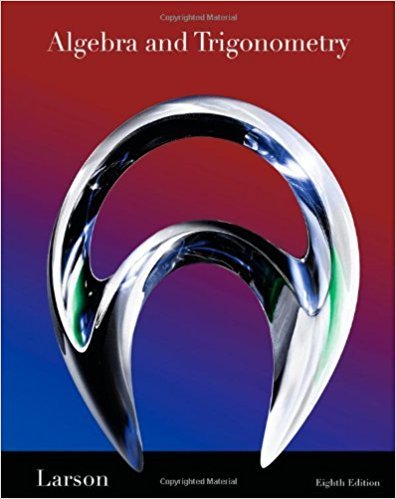×
Get Full Access to Algebra And Trigonometry - 8 Edition - Chapter 9.5 - Problem 43
Get Full Access to Algebra And Trigonometry - 8 Edition - Chapter 9.5 - Problem 43

×

# x2 y x y 7 2 0ISBN: 9781439048474 185

## Solution for problem 43 Chapter 9.5

Algebra and Trigonometry | 8th Edition

• Textbook Solutions
• 2901 Step-by-step solutions solved by professors and subject experts
• Get 24/7 help from StudySoup virtual teaching assistantsAlgebra and Trigonometry | 8th Edition

4 5 1 242 Reviews
30
0
Problem 43

x2 y x y 7 2 0

Step-by-Step Solution:
Step 1 of 3

Math246 Week 8: Complex Roots Now we will look at differential equations with complex roots. This involves equations of the form ay +by +cy=0 . 2 w=κ±γi The roots of the quadratic equation aw +bw+c=0 are (κ+γi)t (−γi)t The general solution is thus y (t=e and y( )=e But this is pretty ugly so we will use Euler’s formula to make it into something nicer. e =cos (θ +isin (θ) −iθ e =cos (−θ +isin (−θ =cos (θ)−isin(θ) So our two general solutions become y(t)=e (cos (γt +isin (γt ) κt y(t)=e (cos (γt −isin (γt)) Now if we add the two equations together and divide by two, we get e cos (γt)

Step 2 of 3

Step 3 of 3

##### ISBN: 9781439048474

Unlock Textbook Solution